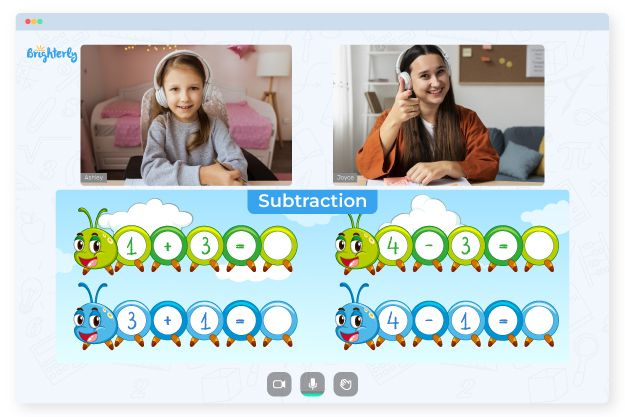# Subtraction Facts Worksheets

Subtraction, just like addition, is a basic, simple, and essential math concept. However, while every kid can get through it, the difficulty level does increase as the numbers do. So, kids need to know how to subtract facts quickly to get through speed tests easily. A way to ensure your kid gets the best math knowledge is by offering them subtraction facts worksheets. Subtraction facts worksheets are sheets that carry over 40 and above subtraction facts questions and answers that a child can practice at home.

Here are some of the benefits of using subtraction math fact worksheets:

### Kids learn to go through subtraction faster

The more facts a kid learns, the easier it will be for them to get through bigger subtraction problems. Then, instead of staying stuck trying to solve for points, they can just remember the ones from the practice tests on basic subtraction facts worksheets.

Math for Kids

Is Your Child Struggling With Math?
1:1 Online Math Tutoring### Teachers get through their curriculum faster

With more kids learning more effectively using subtraction facts worksheets at home, teachers do not have to dwell on a math concept for long because the subtraction math facts worksheets help kids learn the math concepts faster.### Subtraction Facts Worksheet PDF

Basic Subtraction Facts Worksheets### Subtraction Facts Worksheets PDF

Subtraction Facts Worksheets### Subtraction Facts Worksheets PDF

Subtraction Math Facts Worksheet### Subtraction Facts Worksheets PDF

Subtraction Math Fact Worksheets

Struggling with Subtraction?• Does your child struggle with basic subtraction?
• An online tutor could be the solution.

Is your child struggling with the basics of subtraction? Try studying with an online tutor.